## Network Theory (Part 14)

#### John Baez

We've been doing a lot of hard work lately. Let's take a break and think about a fun example from chemistry!

### The ethyl cation

Suppose you start with a molecule of ethane, which has 2 carbons and 6 hydrogens arranged like this:

Then suppose you remove one hydrogen. The result is a positively charged ion, or 'cation'. When I was a kid, I thought the opposite of a cation should be called a 'dogion'. Alas, it's not.

This particular cation, formed from removing one hydrogen from an ethane molecule, is called an 'ethyl cation'. People used to think it looked like this: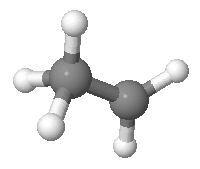They also thought a hydrogen could hop from the carbon with 3 hydrogens attached to it to the carbon with 2. So, they drew a graph with a vertex for each way the hydrogens could be arranged, and an edge for each hop. It looks really cool: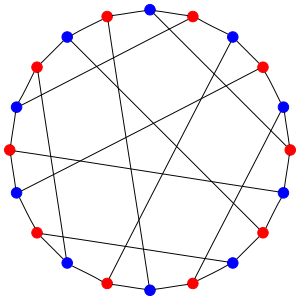The red vertices come from arrangements where the first carbon has 2 hydrogens attached to it, and the blue vertices come from those where the second carbon has 2 hydrogens attached to it. So, each edge goes between a red vertex and a blue vertex.

This graph has 20 vertices, which are arrangements or 'states' of the ethyl cation. It has 30 edges, which are hops or 'transitions'. Let's see why those numbers are right.

First I need to explain the rules of the game. The rules say that the 2 carbon atoms are distinguishable: there's a 'first' one and a 'second' one. The 5 hydrogen atoms are also distinguishable. But, all we care about is which carbon atom each hydrogen is bonded to: we don't care about further details of its location. And we require that 2 of the hydrogens are bonded to one carbon, and 3 to the other.

If you're a physicist, you may wonder why the rules work this way: after all, at a fundamental level, identical particles aren't really distinguishable. I'm afraid I can't give a fully convincing explanation right now: I'm just reporting the rules as they were told to me!

Given these rules, there are 2 choices of which carbon has two hydrogens attached to it. Then there are

$$\displaystyle{ {5 \choose 2} = \frac{5 \times 4}{2 \times 1} = 10}$$

choices of which two hydrogens are attached to it. This gives a total of 2 × 10 = 20 states. These are the vertices of our graph: 10 red and 10 blue.

The edges of the graph are transitions between states. Any hydrogen in the group of 3 can hop over to the group of 2. There are 3 choices for which hydrogen atom makes the jump. So, starting from any vertex in the graph there are 3 edges. This means there are $3 \times 20 / 2 = 30$ edges.

Why divide by 2? Because each edge touches 2 vertices. We have to avoid double-counting them.

### The Desargues graph

The idea of using this graph in chemistry goes back to this paper:

• A. T. Balaban, D. Fărcaşiu and R. Bănică, Graphs of multiple 1,2-shifts in carbonium ions and related systems, Rev. Roum. Chim. 11 (1966), 1205.

This paper is famous because it was the first to use graphs in chemistry to describe molecular transitions, as opposed to using them as pictures of molecules!

But this particular graph was already famous for other reasons. It's called the Desargues-Levi graph, or Desargues graph for short:

Later I'll say why it's called this.

There are lots of nice ways to draw the Desargues graph. For example:

The reason why we can draw such pretty pictures is that the Desargues graph is very symmetrical. Clearly any permutation of the 5 hydrogens acts as a symmetry of the graph, and so does any permutation of the 2 carbons. This gives a symmetry group $S_5 \times S_2$, which has $5! \times 2! = 240$ elements. And in fact this turns out to be the full symmetry group of the Desargues graph.

The Desargues graph, its symmetry group, and its applications to chemistry are discussed here:

• Milan Randic, Symmetry properties of graphs of interest in chemistry: II: Desargues-Levi graph, Int. Jour. Quantum Chem. 15 (1997), 663-682.

### The ethyl cation, revisited

We can try to describe the ethyl cation using probability theory. If at any moment its state corresponds to some vertex of the Desargues graph, and it hops randomly along edges as time goes by, it will trace out a random walk on the Desargues graph. This is a nice example of a Markov process!

We could also try to describe the ethyl cation using quantum mechanics. Then, instead of having a probability of hopping along an edge, it has an amplitude of doing so. But as we've seen, a lot of similar math will still apply.

It should be fun to compare the two approaches. But I bet you're wondering which approach is correct. This is a somewhat tricky question, at least for me. The answer would seem to depend on how much the ethyl cation is interacting with its environment—for example, bouncing off other molecules. When a system is interacting a lot with its environment, a probabilistic approach seems to be more appropriate. The relevant buzzword is 'environmentally induced decoherence'. However, there's something much more basic I have tell you about.

After the paper by Balaban, F.rca.iu and B.nic. came out, people gradually realized that the ethyl cation doesn't really look like the drawing I showed you! It's what chemists call 'nonclassical' ion. What they mean is this: its actual structure is not what you get by taking the traditional ball-and-stick model of an ethane molecule and ripping off a hydrogen. The ethyl cation really looks like this: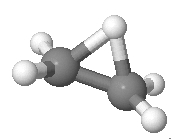For more details, and pictures that you can actually rotate, see:

So, if we stubbornly insist on applying the Desargues graph to realistic chemistry, we need to find some other molecule to apply it to.

### Trigonal bipyramidal molecules

Luckily, there are lots of options! They're called trigonal bipyramidal molecules. They look like this:

The 5 balls on the outside are called 'ligands': they could be atoms or bunches of atoms. In chemistry, 'ligand' just means something that's stuck onto a central thing. For example, in phosphorus pentachloride the ligands are chlorine atoms, all attached to a central phosphorus atom:

It's a colorless solid, but as you might expect, it's pretty nasty stuff: it's not flammable, but it reacts with water or heat to produce toxic chemicals like hydrogen chloride.

Another example is iron pentacarbonyl, where 5 carbon-oxygen ligands are attached to a central iron atom:

You can make this stuff by letting powdered iron react with carbon monoxide. It's a straw-colored liquid with a pungent smell!

Whenever you've got a molecule of this shape, the ligands come in two kinds. There are the 2 'axial' ones, and the 3 'equatorial' ones:

And the molecule has 20 states... but only if count the states a certain way. We have to treat all 5 ligands as distinguishable, but think of two arrangements of them as the same if we can rotate one to get the other. The trigonal bipyramid has a rotational symmetry group with 6 elements. So, there are 5! / 6 = 20 states.

The transitions between states are devilishly tricky. They're called pseudorotations, and they look like this: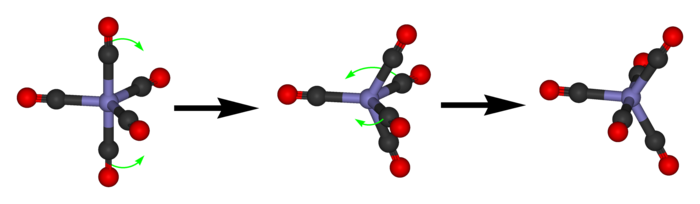If you look very carefully, you'll see what's going on. First the 2 axial ligands move towards each other to become equatorial. Now the equatorial ones are no longer in the horizontal plane: they're in the plane facing us! Then 2 of the 3 equatorial ones swing out to become axial. This fancy dance is called the Berry pseudorotation mechanism.

To get from one state to another this way, we have to pick 2 of the 3 equatorial ligands to swing out and become axial. There are 3 choices here. So, if we draw a graph with states as vertices and transitions as edges, it will have 20 vertices and 20 × 3 / 2 = 30 edges. That sounds suspiciously like the Desargues graph!

Puzzle 1. Show that the graph with states of a trigonal bipyramidal molecule as vertices and pseudorotations as edges is indeed the Desargues graph.

I think this fact was first noticed here:

• Paul C. Lauterbur and Fausto Ramirez, Pseudorotation in trigonal-bipyramidal molecules, J. Am. Chem. Soc. 90 (1968), 6722.6726.

Okay, enough for now! Next time I'll say more about the Markov process or quantum process corresponding to a random walk on the Desargues graph. But since the Berry pseudorotation mechanism is so hard to visualize, I'll pretend that the ethyl cation looks like this:and I'll use this picture to help us think about the Desargues graph.

That's okay: everything we'll figure out can easily be translated to apply to the real-world situation of a trigonal bipyramidal molecule. The virtue of math is that when two situations are 'mathematically the same', or 'isomorphic', we can talk about either one, and the results automatically apply to the other. This is true even if the one we talk about doesn't actually exist in the real world!

### Drawing the Desargues graph

Before we quit for today, let's think a bit more about how we draw the Desargues graph. For this it's probably easiest to go back to our naive model of an ethyl cation:Even though ethyl cations don't really look like this, and we should be talking about some trigonal bipyramidal molecule instead, it won't affect the math to come. Mathematically, the two problems are isomorphic! So let's stick with this nice simple picture.

We can be a bit more abstract, though. A state of the ethyl cation is like having 5 balls, with 3 in one pile and 2 in the other. And we can focus on the first pile and forget the second, because whatever isn't in the first pile must be in the second.

Of course a mathematician calls a pile of things a 'set', and calls those things 'elements'. So let's say we've got a set with 5 elements. Draw a red dot for each 2-element subset, and a blue dot for each 3-element subset. Draw an edge between a red dot and a blue dot whenever the 2-element subset is contained in the 3-element subset. We get the Desargues graph.

That's true by definition. But I never proved that this graph looks like this:

I won't now, either. I'll just leave it as a puzzle:

Puzzle 2. If we define the Desargues graph to have vertices corresponding to 2- and 3-element subsets of a 5-element set, with an edge between vertices when one subset is contained in another, why does it look like the picture above?

To draw a picture we know is correct, it's actually easier to start with a big graph that has vertices for all the subsets of our 5-element set. If we draw an edge whenever an $n$-element subset is contained in an $(n+1)$-element subset, the Desargues graph will be sitting inside this big graph.

This is approximately what the big graph looks like:This graph should have $2^5$ vertices. Unfortunately, some are hidden behind others. It's actually a picture of a 5-dimensional hypercube! The vertices are arranged in columns. There's

• one 0-element subset,
• five 1-element subsets,
• ten 2-element subsets,
• ten 3-element subsets,
• five 4-element subsets,
• one 5-element subset.

So, the numbers of vertices in each column go like this:

$$1 \quad 5 \quad 10 \quad 10 \quad 5 \quad 1$$

which is a row in Pascal's triangle. We get the Desargues graph if we keep only the vertices corresponding to 2- and 3-element subsets, like this: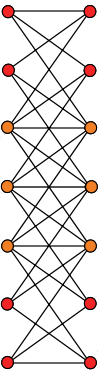Unfortunately, some vertices are hidden behind others, so we don't see 10 vertices in each column, just 7. Laurens Gunnarsen fixed this, and got the following picture of the Desargues graph: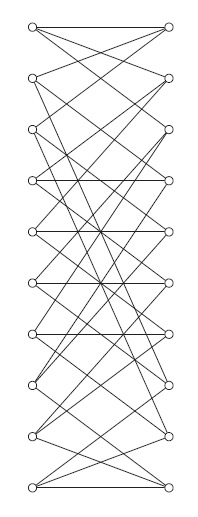It's less pretty than our earlier picture of the Desargues graph, but at least there's no mystery to it. Also, it shows that the Desargues graph can be generalized in various ways. For example, there's a theory of bipartite Kneser graphs $H(n,k).$ The Desargues graph is $H(5,2)$.

### Desargues' theorem

Finally, I can't resist answering this question: why is this graph called the 'Desargues graph'? This name comes from Desargues' theorem, a famous result in projective geometry. Suppose you have two triangles ABC and abc, like this:

Suppose the lines Aa, Bb, and Cc all meet at a single point, the 'center of perspectivity'. Then the point of intersection of ab and AB, the point of intersection of ac and AC, and the point of intersection of bc and BC all lie on a single line, the 'axis of perspectivity'. The converse is true too. Quite amazing!

The Desargues configuration consists of all the actors in this drama:

• 10 points: A, B, C, a, b, c, the center of perspectivity, and the three points on the axis of perspectivity

and

• 10 lines: Aa, Bb, Cc, AB, AC, BC, ab, ac, bc and the axis of perspectivity

Given any configuration of points and lines, we can form a graph called its Levi graph by drawing a vertex for each point or line, and drawing edges to indicate which points lie on which lines. And now for the punchline: the Levi graph of the Desargues configuration is the 'Desargues-Levi graph'!—or Desargues graph, for short.

Alas, I don't know how this is relevant to anything I've discussed. For now it's just a tantalizing curiosity.

I didn't get any solutions of Puzzle 1 the first time I posted this, so I reposed it on Azimuth and the n-Category Café. That brought out lots of great solutions, which I've used to compose the answer here. I'm especially indebted to J.M. Allen, Twan van Laarhoven, Peter Morgan and Johan Swanljung.

Puzzle 1. Show that the graph with states of a trigonal bipyramidal molecule as vertices and pseudorotations as edges is indeed the Desargues graph.

Answer. To be specific, let's use iron pentacarbonyl as our example of a trigonal bipyramidal molecule: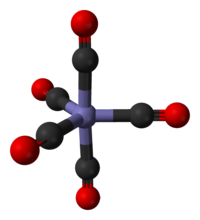It suffices to construct a 1-1 correspondence between the states of this molecule and those of the ethyl cation, such that two states of this molecule are connected by a transition if and only if the same holds for the corresponding states of the ethyl cation.

Here's the key idea: the ethyl cation has 5 hydrogens, with 2 attached to one carbon and 3 attached to the other. Similarly, the trigonal bipyramidal molecule has 5 carbonyl grops, with 2 axial and 3 equatorial. We'll use this resemblance to set up our correspondence.

There are various ways to describe states of the ethyl cation, but this is the best for us. Number the hydrogens 1,2,3,4,5. Then a state of the ethyl cation consists of a partition of the set {1,2,3,4,5} into a 2-element set and a 3-element set, together with one extra bit of information, saying which carbon has 2 hydrogens attached to it. This extra bit is the color here:What do transitions look like in this description? When a transition occurs, two hydrogens that belonged to the 3-element set now become part of the 2-element set. Meanwhile, both hydrogens that belonged to the 2-element set now become part of the 3-element set. (Ironically, the one hydrogen that hops is the one that stays in the 3-element set.) Moreover, the extra bit of information changes. That's why every edge goes from a red dot to a blue one, or vice versa.

So, to solve the puzzle, we need to show that the same description also works for the states and transitions of iron pentacarbonyl!

In other words, we need to describe its states as ways of partitioning the set {1,2,3,4,5} into a 2-element set and a 3-element set, together with one extra bit of information. And we need its transitions to switch two elements of the 2-element set with two of the 3-element set, while changing that extra bit.

To do this, number the carbonyl groups 1,2,3,4,5. The 2-element set consists of the axial ones, while the 3-element set consists of the equatorial ones. When a transition occurs, two of axial ones trade places with two of the equatorial ones, like this:So, now we just need to figure out what that extra bit of information is, and why it always changes when a transition occurs.

Here's how we calculate that extra bit. Hold the iron pentacarbonyl molecule vertically with one of the equatorial carbonyl groups pointing to your left. Remember, the carbonyl groups are numbered. So, write a list of these numbers, say (a,b,c,d,e), where a is the top axial one, b,c,d are the equatorial ones listed in counterclockwise order starting from the one pointing left, and e is the bottom axial one. This list is some permutation of the list (1,2,3,4,5). Take the sign of this permutation to be our bit!

Let's do an example: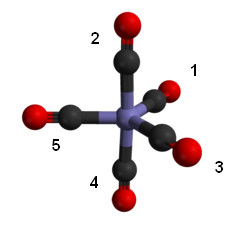Here we get the list (2,5,3,1,4) since 2 is on top, 4 is on bottom, and 5,3,1 are the equatorial guys listed counterclockwise starting from the one at left. The list (2,5,3,1,4) is an odd permutation of (1,2,3,4,5), so our bit of information is odd.

Of course we must check that this bit is well-defined: namely, that it doesn't change if we rotate the molecule. Rotating it a third of a turn gives an even permutation of the equatorial guys and leaves the axial ones alone, so this is an even permutation. Flipping it over gives an odd permutation of the equatorial guys, but it also gives an odd permutation of the axial ones, so this too is an even permutation. So, rotating the molecule doesn't change the sign of the permutation we compute from it. The sign is thus a well-defined function of the state of the molecule.

Next we must to check that this sign changes whenever our molecule undergoes a transition. For this we need to check that any transition changes our list of numbers by an odd permutation. Since all transitions are conjugate in the permutation group, it suffices to consider one example: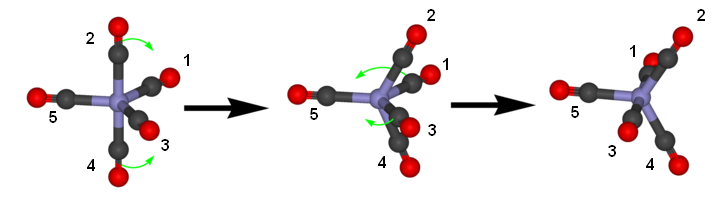Here we started with a state giving the list (2,5,3,1,4). The transition ttakes us to a state that gives the list (3,5,4,2,1) if we hold the molecule so that 3 is pointing up and 5 to the left. The reader can check that going from one list to another requires an odd permutation. So we're done.   █

Tracy Hall's discussion of the above puzzle was very interesting and erudite. He also addressed an interesting subsidiary puzzle, namely: what graph do we get if we discard that extra bit of information that says which carbon in the ethyl cation has 3 hydrogens attached to it and which has 2? The answer is the Petersen graph:Tracy wrote:

As some comments have pointed out over on Azimuth, in both cases there are ten underlying states which simply pick out two of the five pendant atoms as special, together with an extra parity bit (which can take either value for any of the ten states), giving twenty states in total. The correspondence of the ten states is clear: an edge exists between state A and state B, in either case, if and only if the two special atoms of state A are disjoint from the two special atoms of state B. This is precisely one definition of the Petersen graph (a famous 3-valent graph on 10 vertices that shows up as a small counterexample to lots of naove conjectures). Thus the graph in either case is a double cover of the Petersen graph—but that does not uniquely specify it, since, for example, both the Desargues graph and the dodecahedron graph are double covers of the Petersen graph.

For a labeled graph, each double cover corresponds uniquely to an element of the $\mathbb{Z}/2\mathbb{Z}$ cohomology of the graph (for an unlabeled graph, some of the double covers defined in this way may turn out to be isomorphic). Cohomology over $\mathbb{Z}/2\mathbb{Z}$ takes any cycle as input and returns either 0 or 1, in a consistent way (the output of a $\mathbb{Z}/2\mathbb{Z}$ sum of cycles is the sum of the outputs on each cycle). The double cover has two copies of everything in the base (Petersen) graph, and as you follow all the way around a cycle in the base, the element of cohomology tells you whether you come back to the same copy (for 0) or the other copy (for 1) in the double cover, compared to where you started.

One well-defined double cover for any graph is the one which simply switches copies for every single edge (this corresponding to the element of cohomology which is 1 on all odd cycles and 0 on all even cycles). This always gives a double cover which is a bipartite graph, and which is connected if and only if the base graph is connected and not bipartite. So if we can show that in both cases (the fictitious ethyl cation and phosphorus pentachloride) the extra parity bit can be defined in such a way that it switches on every transition, that will show that we get the Desargues graph in both cases.

The fictitious ethyl cation is easy: the parity bit records which carbon is which, so we can define it as saying which carbon has three neighbors. This switches on every transition, so we are done. Phosphorus pentachloride is a bit trickier; the parity bit distinguishes a labeled molecule from its mirror image, or enantiomer. As has already been pointed out on both sites, we can use the parity of a permutation to distinguish this, since it happens that the orientation-preserving rotations of the molecule, generated by a three-fold rotation acting as a three-cycle and by a two-fold rotation acting as a pair of two-cycles, are all even permutations, while the mirror image that switches only the two special atoms is an odd permutation. The pseudorotation can be followed by a quarter turn to return the five chlorine atoms to the five places previously occupied by chlorine atoms, which makes it act as a four-cycle, an odd permutation. Since the parity bit in this case also can be defined in such a way that it switches on every transition, the particular double cover in each case is the Desargues graph—a graph I was surprised to come across here, since just this past week I have been working out some combinatorial matrix theory for the same graph!

The five chlorine atoms in phosphorus pentachloride lie in six triangles which give a triangulation of the 2-sphere, and another way of thinking of the pseudorotation is that it corresponds to a Pachner move or bistellar flip on this triangulation—in particular, any bistellar flip on this triangulation that preserves the number of triangles and the property that all vertices in the triangulation have degree at least three corresponds to a pseudorotation as described.

The next puzzle was both posed and answered by Greg Egan on Azimuth.

Puzzle 2. If we define the Desargues graph to have vertices corresponding to 2- and 3-element subsets of a 5-element set, with an edge between vertices when one subset is contained in another, why does it look like this picture?Answer. For $i=0,...,4$, and with all the additions below modulo 5, define five pairs of red dots as: $$\{i, i+1\}, \{i+1,i+4\}$$ and five pairs of blue dots as: $\{i, i+1, i+4\}, \{i+1, i+2, i+4\}$ The union of the $i$th pair of red dots is the first of the $i$th pair of blue dots, and the union of the second of the $i$th pair of red dots and the first of the $(i+1)$th pair of red dots is the second of the $i$th pair of blue dots. So if we form a 20-sided polygon whose vertices are alternating red and blue dots generated in this order, all the edges of the polygon will join red dots to blue dots of which they are subsets: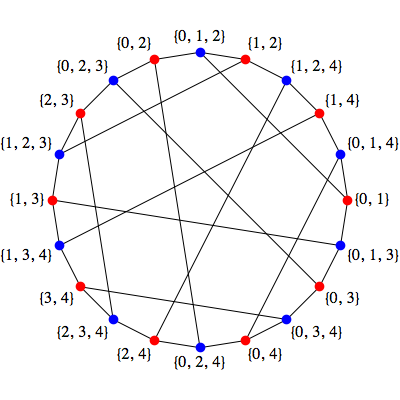The first red dot of the $i$th pair is also a subset of the first blue dot of the $(i+1)$th pair:

$$\{i+1, i+2, i\}$$

which gives the five short chords in the picture, while the second red dot of the $i$th pair is a subset of the second blue dot of the $(i+2)$ pair:

$$\{i+3, i+4, i+1\}$$

which gives the five long chords in the picture.   █